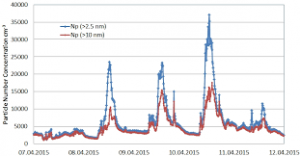## How to Calculate and Solve for the Number of Entities of a Constituent, Volume and Number Concentration in Chemistry | Nickzom CalculatorThe image above represents the number concentration.

To compute for the number concentration, two essential parameters are needed and these parameters are Number of Entities of the Constituent (N) and Volume (V).

The formula for calculating the number concentration:

C = NV

Where;
C = Number Concentration
N = Number of Entities of the Constituent
V = Volume

Let’s solve an example;
Find the number concentration when the number of entities of the constituent is 12 and the volume is 24.

This implies that;
N = Number of Entities of the Constituent = 12
V = Volume = 24

C = NV
C = 1224
C = 0.5

Therefore, the number concentration is 0.5 m-3.

Calculating the Number of Entities of the Constituent when Number Concentration and Volume is Given.

N = VC

Where;
N = Number of Entities of the Constituent
C = Number Concentration
V = Volume

Let’s solve an example;
Find the number of entities of the constituent when the number concentration is 18 and the volume is 14.

This implies that;
C = Number Concentration = 18
V = Volume = 14

N = VC
N = 14 x 18
N = 252

Therefore, the number of entities of the constituent is 252 m-1.